Documentation

# imdiffusefilt

Anisotropic diffusion filtering of images

## Syntax

``J = imdiffusefilt(I)``
``J = imdiffusefilt(I,Name,Value)``

## Description

example

````J = imdiffusefilt(I)` applies anisotropic diffusion filtering to image `I` and returns the result in `J`.```

example

````J = imdiffusefilt(I,Name,Value)` uses name-value pairs to change the behavior of the anisotropic diffusion algorithm.```

## Examples

collapse all

Read an image into the workspace and display it.

```I = imread('cameraman.tif'); imshow(I) title('Original Image')```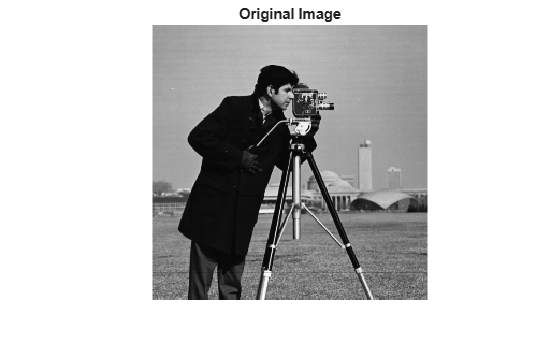Smooth the image using anisotropic diffusion. For comparison, also smooth the image using Gaussian blurring. Adjust the standard deviation `sigma` of the Gaussian smoothing kernel so that textured regions, such as the grass, are smoothed a similar amount for both methods.

```Idiffusion = imdiffusefilt(I); sigma = 1.2; Igaussian = imgaussfilt(I,sigma);```

Display the results.

```montage({Idiffusion,Igaussian},'ThumbnailSize',[]) title('Smoothing Using Anisotropic Diffusion (Left) vs. Gaussian Blurring (Right)')```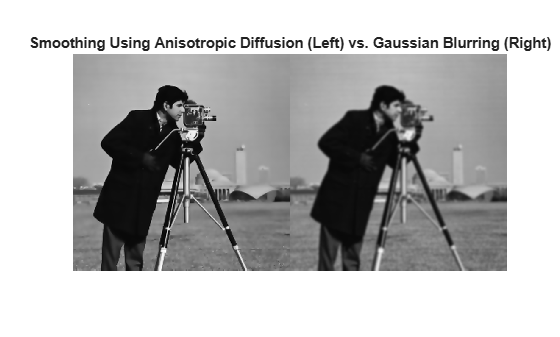Anisotropic diffusion preserves the sharpness of edges better than Gaussian blurring.

Read a grayscale image, then apply strong Gaussian noise to it. Display the noisy image.

```I = imread('pout.tif'); noisyImage = imnoise(I,'gaussian',0,0.005); imshow(noisyImage) title('Noisy Image')```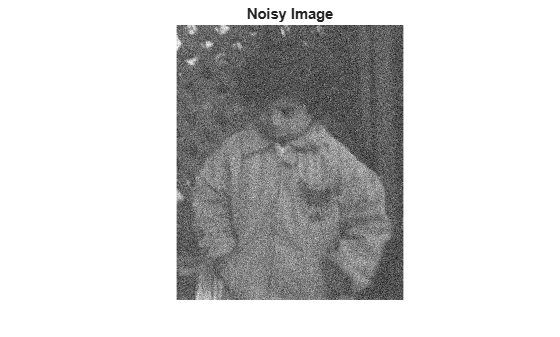Compute the structural similarity index (SSIM) to measure the quality of the noisy image. The closer the SSIM value is to 1, the better the image agrees with the noiseless reference image.

```n = ssim(I,noisyImage); disp(['The SSIM value of the noisy image is ',num2str(n),'.'])```
```The SSIM value of the noisy image is 0.26556. ```

Reduce the noise using anisotropic diffusion. First, try the default parameters for the anisotropic diffusion filter, and display the result.

```B = imdiffusefilt(noisyImage); imshow(B) title('Anisotropic Diffusion with Default Parameters')```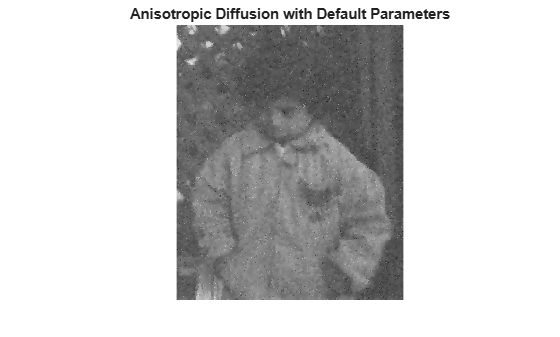```nB = ssim(I,B); disp(['The SSIM value using default anisotropic diffusion is ',num2str(nB),'.'])```
```The SSIM value using default anisotropic diffusion is 0.65665. ```

The image is still degraded by noise, so refine the filter. Choose the quadratic conduction method because the image is characterized more by wide homogenous regions than by high-contrast edges. Estimate the optimal gradient threshold and number of iterations by using the `imdiffuseest` function. Display the resulting image.

```[gradThresh,numIter] = imdiffuseest(noisyImage,'ConductionMethod','quadratic'); C = imdiffusefilt(noisyImage,'ConductionMethod','quadratic', ... 'GradientThreshold',gradThresh,'NumberOfIterations',numIter); imshow(C) title('Anisotropic Diffusion with Estimated Parameters')```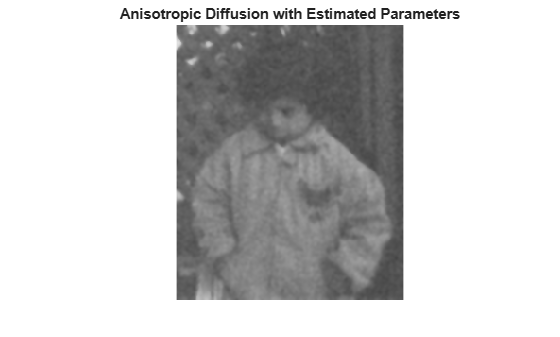```nC = ssim(I,C); disp(['The SSIM value using quadratic anisotropic diffusion is ',num2str(nC),'.'])```
```The SSIM value using quadratic anisotropic diffusion is 0.88135. ```

Noise is less apparent in the resulting image. The SSIM value, which is closer to 1, confirms that the quality of the image has improved.

Load a noisy 3-D grayscale MRI volume.

`load mristack`

Perform edge-aware noise reduction on the volume using anisotropic diffusion. To prevent over-smoothing the low-contrast features in the brain, decrease the number of iterations from the default number, 5. The tradeoff is that less noise is removed.

`diffusedImage = imdiffusefilt(mristack,'NumberOfIterations',3);`

To compare the noisy image and the filtered image in detail, display the tenth slice of both.

```imshowpair(mristack(:,:,10),diffusedImage(:,:,10),'montage') title('Noisy Image (Left) vs. Anisotropic-Diffusion-Filtered Image (Right)')```Calculate the Naturalness Image Quality Evaluator (NIQE) score averaged over all slices in the volume. The NIQE score provides a quantitative measure of image quality that does not require a reference image. Lower NIQE scores reflect better perceptual image quality.

```nframes = size(mristack,3); m = 0; d = 0; for i = 1:nframes m = m + niqe(mristack(:,:,i)); d = d + niqe(diffusedImage(:,:,i)); end mAvg = m/nframes; dAvg = d/nframes; disp(['The NIQE score of the noisy volume is ',num2str(mAvg),'.'])```
```The NIQE score of the noisy volume is 5.7794. ```
`disp(['The NIQE score using anisotropic diffusion is ',num2str(dAvg),'.'])`
```The NIQE score using anisotropic diffusion is 4.1391. ```

The NIQE score is consistent with the observation of reduced noise in the filtered image.

## Input Arguments

collapse all

Image to filter, specified as a 2-D grayscale image of size m-by-n or a 3-D grayscale volume of size m-by-n-by-k.

### Note

To apply anisotropic diffusion filtering to a color image, use `imdiffusefilt` on each color channel independently.

Data Types: `single` | `double` | `int8` | `int16` | `int32` | `uint8` | `uint16` | `uint32`

### Name-Value Pair Arguments

Specify optional comma-separated pairs of `Name,Value` arguments. `Name` is the argument name and `Value` is the corresponding value. `Name` must appear inside quotes. You can specify several name and value pair arguments in any order as `Name1,Value1,...,NameN,ValueN`.

Example: `imdiffusefilt(I,'NumberOfIterations',4,'Connectivity','minimal')` performs anisotropic diffusion on image `I`, using 4 iterations and minimal connectivity.

Gradient threshold, specified as the comma-separated pair consisting of `'GradientThreshold'` and a numeric scalar or a numeric vector of length `NumberOfIterations`. The value of `GradientThreshold` controls the conduction process by classifying gradient values as an actual edge or as noise. Increasing the value of `GradientThreshold` smooths the image more. The default value is 10% of the dynamic range of the image. You can use the `imdiffuseest` function to estimate a suitable value of `GradientThreshold`.

Number of iterations to use in the diffusion process, specified as the comma-separated pair consisting of `'NumberOfIterations'` and a positive integer. You can use the `imdiffuseest` function to estimate a suitable value of `NumberOfIterations`.

Connectivity of a pixel to its neighbors, specified as the comma-separated pair consisting of `'Connectivity'` and one of these values:

• `'maximal'` — Considers 8 nearest neighbors for 2-D images, and 26 nearest neighbors for 3-D images

• `'minimal'` — Considers 4 nearest neighbors for 2-D images, and 6 nearest neighbors for 3-D images

Conduction method, specified as the comma-separated pair consisting of `'ConductionMethod'` and `'exponential'` or `'quadratic'`. Exponential diffusion favors high-contrast edges over low-contrast edges. Quadratic diffusion favors wide regions over smaller regions.

## Output Arguments

collapse all

Diffusion-filtered image, returned as a numeric array of the same size and data type as the input image, `I`.

 Perona, P., and J. Malik. "Scale-space and edge detection using anisotropic diffusion." IEEE® Transactions on Pattern Analysis and Machine Intelligence. Vol. 12, No. 7, July 1990, pp. 629–639.

 Gerig, G., O. Kubler, R. Kikinis, and F. A. Jolesz. "Nonlinear anisotropic filtering of MRI data." IEEE Transactions on Medical Imaging. Vol. 11, No. 2, June 1992, pp. 221–232.